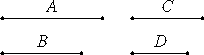# Proposition 11

If four magnitudes are proportional, and the first is commensurable with the second, then the third also is commensurable with the fourth; but, if the first is incommensurable with the second, then the third also is incommensurable with the fourth.

Let A, B, C, and D be four magnitudes in proportion, so that A is to B as C is to D, and let A be commensurable with B.

I say that C is also commensurable with D.X.5

Since A is commensurable with B, therefore A has to B the ratio which a number has to a number.

And A is to B as C is to D, therefore C also has to D the ratio which a number has to a number. Therefore C is commensurable with D.

Next, let A be incommensurable with B.

I say that C is also incommensurable with D.

X.7

Since A is incommensurable with B, therefore A does not have to B the ratio which a number has to a number.

And A is to B as C is to D, therefore neither has C to D the ratio which a number has to a number. Therefore C is incommensurable with D.

Therefore, if four magnitudes are proportional, and the first is commensurable with the second, then the third also is commensurable with the fourth; but, if the first is incommensurable with the second, then the third also is incommensurable with the fourth.

Q.E.D.

## Guide

The proof if very direct. If A : B = C : D, and the first ratio equals a numeric ratio, then the second equals that, too, but if the first is not a numeric ratio, then neither is the second.

This proposition is used in repeatedly in Book X starting with X.14. It is also used in the previous proposition which was, no doubt, not in the original Elements.